# Peirce's law

Peirce's law is a formula in propositional calculus that is commonly expressed in the following form: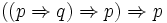Peirce's law holds in classical propositional calculus, but not in intuitionistic propositional calculus. The precise axiom system that one chooses for classical propositional calculus determines whether Peirce's law is taken as an axiom or proven as a theorem.

## History

Here is Peirce's own statement and proof of the law:

 A fifth icon is required for the principle of excluded middle and other propositions connected with it. One of the simplest formulae of this kind is:This is hardly axiomatical. That it is true appears as follows. It can only be false by the final consequent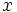being false while its antecedent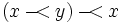is true. If this is true, either its consequent,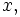is true, when the whole formula would be true, or its antecedent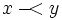is false. But in the last case the antecedent ofthat ismust be true. (Peirce, CP 3.384).

Peirce goes on to point out an immediate application of the law:

 From the formula just given, we at once get: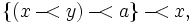where the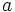is used in such a sense thatmeans that from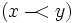every proposition follows. With that understanding, the formula states the principle of excluded middle, that from the falsity of the denial offollows the truth of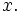(Peirce, CP 3.384).

Note. Peirce uses the sign of illation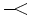” for implication. In one place he explains “” as a variant of the sign “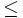” for less than or equal to; in another place he suggests that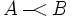is an iconic way of representing a state of affairs where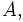in every way that it can be, is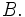## Graphical proof

Under the existential interpretation of Peirce's logical graphs, Peirce's law is represented by means of the following formal equivalence or logical equation.

Proof. Using the axiom set given in the entry for logical graphs, Peirce's law may be proved in the following manner.

The following animation replays the steps of the proof.

## Equational form

A stronger form of Peirce's law also holds, in which the final implication is observed to be reversible: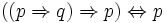### Proof 1

Given what precedes, it remains to show that: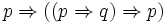But this is immediate, since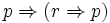for any proposition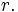### Proof 2

Representing propositions as logical graphs under the existential interpretation, the strong form of Peirce's law is expressed by the following equation:

Using the axioms and theorems listed in the article on logical graphs, the equational form of Peirce's law may be proved in the following manner:

The following animation replays the steps of the proof.

## Bibliography

• Peirce, Charles Sanders (1885), "On the Algebra of Logic : A Contribution to the Philosophy of Notation", American Journal of Mathematics 7 (1885), 180–202. Reprinted (CP 3.359–403), (CE 5, 162–190).
• Peirce, Charles Sanders (1931–1935, 1958), Collected Papers of Charles Sanders Peirce, vols. 1–6, Charles Hartshorne and Paul Weiss (eds.), vols. 7–8, Arthur W. Burks (ed.), Harvard University Press, Cambridge, MA. Cited as (CP volume.paragraph).
• Peirce, Charles Sanders (1981–), Writings of Charles S. Peirce : A Chronological Edition, Peirce Edition Project (eds.), Indiana University Press, Bloomington and Indianapolis, IN. Cited as (CE volume, page).

## Document history

Portions of the above article were adapted from the following sources under the GNU Free Documentation License, under other applicable licenses, or by permission of the copyright holders.